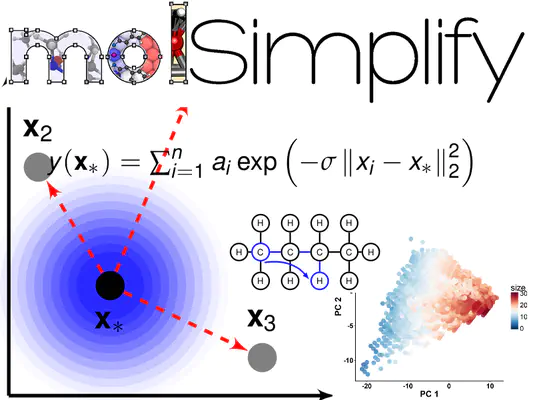# QM9 kernel models using molSimplify, RACs and R: Part 1In this two-part tutorial, we’ll show you how to use molSimplify to collect autocorrelation-based descriptors from molecular structures and use those to make predictions using a simple kernel ridge regression (KRR) model, as shown in our recent paper. In this first part, we will explain how to use python and molSimplify to extract the molecular descriptors and get our data ready for the learning task. We will also provide a copy of the output of the preprocessing step if you want to proceed straight the learning a model in the second tutorial. The first thing we need is some data to train on. One of the most common test sets used in atomistic machine learning is the QM9 dataset from Ramarkrishnan et. al (2014) , consisting of about 134,000 small CHONF organic molecules. The approach outlined here will work with minor modifications for any other set of organic molecules provided as xyz files, and extension to inorganic complexes is easily achieved.

We’ll begin by downloading the data from figshare. The data is available as a zipped folder, so unzip it and rename the `dsgdb9nsd.xyz` folder `qm9_data`. NOTE: the folder, as it is provided from figshare, has a “.xyz” in the name - and some operating systems will interpret this as a file extension, and the rename will not work correctly. We recommend renaming the folder from the console using mv or equivalent. Once we’ve unzipped the folder, we find a that the data is stored in a customized xyz format, containing various atomic properties, 3D coordinates of the DFT-optimized molecule, as well as the SMILES and InChl strings for each case. The first step is to convert this data into a set of standard xyz files the molSimplify can understand, as well as extract the atomization energy, which we will use as the target of regression model (simple variations will allow you to extract the other 14 DFT properties from the file, but we’ll keep it simple for now). Since the atomization energy is the quantity we are predicting, but the data supplies the absolute internal energy, we’ll need to correct for the reference atomic energies given in the file `atomref.txt`. For each molecule, we need to subtract the correct number of atomic reference terms from the given internal energy (U0), for example for CH2OH, we’d need to subtract the energy of C, O and 3 Hs. For convenience, we’ll do this at the same time as reading the data. We’ll also grab and tabulate the SMILES strings so we can ID the molecules easily. We have provided a simple script, qm9_preprocess.py, that will accomplish this process. To work out the box, you will need an installation of molSimplify and place the folder `qm9_data`, the file “atomref.txt” from figshare, and the script qm9_preprocess.py in the same folder. The provided script has some boilerplate text extraction steps that we won’t discuss, but we’ll take a look at some of the key lines:

``````from molSimplify.Informatics.autocorrelation import generate_full_complex_autocorrelations
from molSimplify.Classes.mol3D import mol3D
``````

These lines load molSimplify informatics functions and the mol3D class into the current python working environment and will provide us with methods to extract features for the data. Next, we will loop over each geometry, and extract a canonical xyz file by trimming off the extra information and saving this in a new folder named `qm9_geos`. As we copy the coordinates over, we’ll make a note of how many atoms of each type are present and add the total atomization energy correction needed, which we’ll get from a dictionary `atom_u0_dict` built from the `atomref.txt` file:

``````if (i < (natoms +2)) and not (i==1):
newf.write(lines)
this_atom = lines.split()
this_correction += -1*float(atom_u0_dict[this_atom])
``````

At the same time, we will mine the text for the U0 energy, which is the 12th element of the (space delimited) second line of the file, and add in our correction above. After this is done, create a `mol3D` object for each complex:

``````this_mol = mol3D()
``````

The `mol3D` object is the central working part of molSimplify, and most of the functions provided will take inputs of `mol3D` class. For example, to calculate the depth-0 to depth-3 full structure RACs for a given `mol3D` object, we use:

``````results_dictionary = generate_full_complex_autocorrelations(self.mol,depth=3,loud=False)
``````

here, “loud” will determine if diagnostic output should be printed to standard out, but with the large number of molecules we are processing it is better left off. The rest of the script will write csv files containing the u0 values and RACs, and we shan’t dwell on them further.

Since we are looping over 134k molecules and extracting standard geometries for each one, the processing of these files might take a while, depending on your configuration. It takes around 2 minutes on our workstation. We have supplied QM9_descriptor_file.csv, which contains the autocorrelations and QM9_smiles.csv which contains the SMILES strings. These are the outputs of the qm9_preprocess.py script.

We will make a final important comment that the use of the optimized geometry is not needed as the RAC function only encode connectivity information – in this case, it is convenient enough to use the built in function since we have the geometries. It would be possible to convert from SMILEs strings directly to RACs but in this case the data is supplied as individual geometry files already.